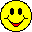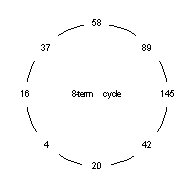# Happy & Dizzy Numbers## Part I: Happy Numbers

### INTRODUCTION

젨젨잹efore we can explain what a happy number is, you have to learn a new idea, called "recurrent operations." As the word "recur" means "to happen again", a recurrent operation must mean a mathematical procedure that is repeated. A very simple example would be the rule "add 5 to the result". If we started with the number 0 and applied that recurrent operation rule, we would produce the sequence 0, 5, 10, 15, 20, ... ; this list is the famous "multiples of five".

젨젨쟏f course, there are all kinds of recurrent operation rules in mathematics. Another important rule is "multiply each result by 2". If we used 1 as our first number, this sequence shows up: 1, 2, 4, 8, 16, 32, ... ; this list is the also famous "powers of two". So, you see it's really not such a difficult idea now, is it?

젨젨쟄owever, in order to produce "happy numbers", we will invent a rule that is just a little bit more complicated. (After all, you didn't expect this to be that easy, did you?) Our rule now will be given in two steps: (1) find the squares of the digits of the starting number; then (2) add those squares to get the result that will be used in the repeat part of your work.

32 + 72 + 52 = 9 + 49 + 25 = 83

젨젨쟍ow we repeat the R.O. procedure with 83. This gives us:

82 + 32 = 64 + 9 = 73

젨젨쟏f course, we continue with 73. This will produce 58.

### HAPPY NUMBERS

젨젨잹ut we can hear you saying: "When do I stop? What's the point of all this?" That's the beautiful part of the story. The answer is: when you see something strange happening. The strange thing that tells when a number is happy is simply this: the result of a 1 eventually occurs. Here is an example, starting with the number 23:

4 + 9 = 13; 1 + 9 = 10; and 1 + 0 = 1.

It's that easy! When you reach a 1, the starting number is called happy. [But don't ask why it's happy, instead of sad; that's just what the books say.]

젨젨쟏nce you determine a number is happy, you can say all the intermediate results are also happy. The numbers 13 and 10 must also be considered as happy, because they too produce a 1.

젨젨잺an you find some more happy numbers? Yes. If you know a certain number is happy, it's easy to find many more. How? One way is to insert a zero or two. Look: above we saw that 23 was happy, right? This means that 203 is also happy; so is 230. A larger example is 2003. See? Now you can make many, many happy numbers, using an old one with as many zeros as you wish.

젨젨잹ut that's the easy way. You want something a bit more challenging, don't you? Well, that's your task now -- find some more happy numbers without using the "zeros" technique. Okay?

### Part II: Dizzy Numbers

젨젨쟕he term "dizzy numbers" was invented by me. It is based on an idea that should occur to anyone searching for happy numbers, because often they find themselves "going in circles", literally, i.e. getting dizzy. Here's why:젨젨쟓ecall the number 375 from above? It produced the sequence 83, 73, 58,... But we stopped there in our explanation of the RO procedure. If we had continued, we would have had 89, 145, 42, 20, 4, 16, 37, and then back to 58! Hmm... now that's strange, isn't it? We've returned to where we were (58) just eight steps earlier; we've gone in a circle. We've produced an 8-term numerical cycle. Hence, we're getting a little dizzy. (Get it?)

젨젨쟔o we can now define more formally a dizzy number to be one that is either part of that cycle or produces a sequence that enters the cycle eventually (like 375 did).

젨젨쟍ow, do you want to hear something really strange? All numbers that are not happy are dizzy! That's right. No matter how big or small a number may be, if you use the sum-of-the-squares-of-the-digits RO procedure on it, you either reach a 1 or the 8-term cycle. Amazing, isn't it?

젨젨쟍ow armed with this new knowledge, you are ready to classify any number as happy or dizzy.

젨젨쟄ave fun!

For more activities about recurrent operations, go to Kaprekar or Ulam.